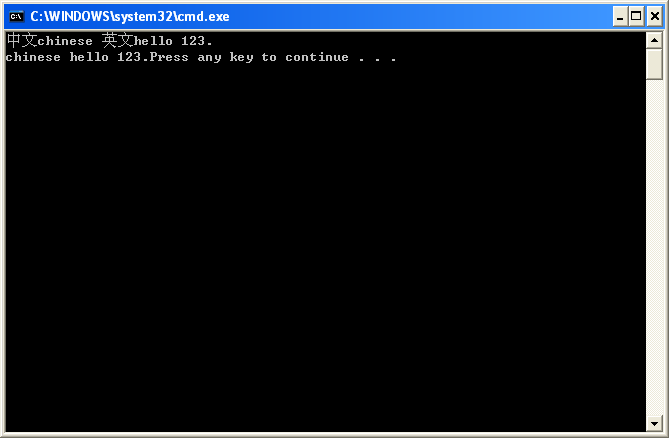2020-05-17 16:47

# 请问如何实现利用指针方式实现将一个字符串中的非英文字符删除，并输出新字符串。(C语言）

C语言：利用指针方式实现将一个字符串中的非英文字符删除，并输出新字符串。

• 写回答
• 好问题 提建议
• 追加酬金
• 关注问题
• 邀请回答

#### 2条回答默认 最新

•threenewbee 2020-05-18 06:36
最佳回答

非英文字符定义不明确，是删除非英文字母，还是删除非英文语言的字符（比如删除中文，保留英文数字、字母、符号）
我的代码是后者，如果你要前者，if (s[i] > 0) 修改为 if ((s[i] >= 'a' && s[i] <= 'z') || (s[i] >= 'A' && s[i] <= 'Z'))

# 问题解决的话，请点采纳

``````#include <stdio.h>

void delNoneEnglishChar(char * s)
{
int i, j;
for (i = j = 0; s[i]; i++)
{
if (s[i] > 0)
s[j++] = s[i];
}
s[j] = 0;
}
int main()
{
char ch;
scanf("%[^\n]", &ch);
delNoneEnglishChar(ch);
printf("%s", ch);
return 0;
}
``````评论
解决 3 无用
打赏 举报